﻿ Some Iterative Methods for Solving Nonlinear Equations

Some Iterative Methods for Solving Nonlinear Equations

Rostam K. Saeed, Karwan H.F.Jwamer, Delan O. Salem

American Journal of Numerical Analysis

Some Iterative Methods for Solving Nonlinear Equations

Rostam K. Saeed1,, Karwan H.F.Jwamer2, Delan O. Salem1

1Department of Mathematics, College of Science-Salahaddin University/Erbil, Halwer-Kurdistan Region, Iraq

2Department of Mathematics, School of Science -Sulaimani University, Sulaimani -Kurdistan Region, Iraq

Abstract

In this paper, three iteration methods are introduced to solve nonlinear equations. The convergence criteria for these methods are also discussed. Several examples are presented and compared to other well-known methods, showing the accuracy and fast convergence of the proposed methods.

• Rostam K. Saeed, Karwan H.F.Jwamer, Delan O. Salem. Some Iterative Methods for Solving Nonlinear Equations. American Journal of Numerical Analysis. Vol. 3, No. 2, 2015, pp 49-51. http://pubs.sciepub.com/ajna/3/2/3
• Saeed, Rostam K., Karwan H.F.Jwamer, and Delan O. Salem. "Some Iterative Methods for Solving Nonlinear Equations." American Journal of Numerical Analysis 3.2 (2015): 49-51.
• Saeed, R. K. , H.F.Jwamer, K. , & Salem, D. O. (2015). Some Iterative Methods for Solving Nonlinear Equations. American Journal of Numerical Analysis, 3(2), 49-51.
• Saeed, Rostam K., Karwan H.F.Jwamer, and Delan O. Salem. "Some Iterative Methods for Solving Nonlinear Equations." American Journal of Numerical Analysis 3, no. 2 (2015): 49-51.

 Import into BibTeX Import into EndNote Import into RefMan Import into RefWorks

1. Introduction

One of the oldest and most basic problems in mathematics is that of solving an nonlinear equation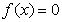. This problem has motivated many theoretical developments including the fact that solution formulas do not in general exist.

Thus, the development of algorithms for finding solution has historically been an important enterprise. Newton-Raphson method  is the most popular technique for solving nonlinear equations. Many topics related to Newton ̓s method still attract attention from researchers. As is well known, a disadvantage of the method is that the initial approximation, must be chosen sufficiently close to a true solution in order to guarantee their convergence.

Finding a criterion for choosing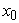is quite difficult and difficult and therefore effective and globally convergent algorithms are needed. In recent years, several methods have been developed to solve the nonlinear equation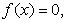by Newton method and their modifications [2,4-11]. Supposeis a sequence that convergent towith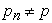for allIf positive constants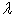and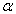exist with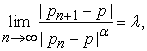then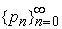converges toof order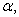with asymptotic error constant. In general, a sequence with a high order of convergence more rapidly thansequence with a lower order.

In this work, a cubic iterative methods based on Taylor ̓s series expansion are introduced as follows:

Consider a nonlinear equationThe Taylor ̓s series expansion around a given initial pointassumingbeing close enough to the simple root, is given as follows:(1)

where HOT denotes the higher order terms. Then the nonlinear equation becomes,(2)

when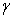is close enough toequation (2) can be approximated as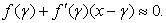(3)

Thus we have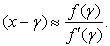(4)

By assumingwhich yields the one-step iteration method called Newton method  with second-order convergence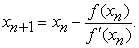(5)

2. New Iterative Methods

Depending on the relations (1)-(5), a new one-step iteration method can be constructed with third-order convergence. For this reason, we rewrite equation (2) as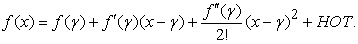(6)

Equation (6) can be approximated as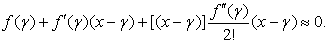(7)

Substituting (4) into the bracket of equation (7),

We obtain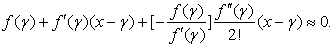That is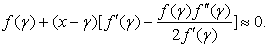(8)

From equation (8),we obtain(9)

Thus we can solve equation (9) by assumingas(10)

This suggests that following one-step iteration method:

Algorithm 1.1: For a givencompute the approximate solutionby the one-step iteration scheme:(11)

It will be shown that the proposed method (11) has third order convergence, and this will be done by applying the following Maple13 program: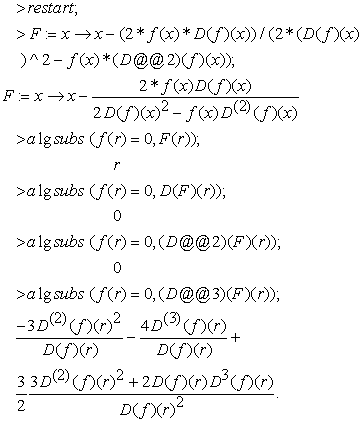To derive another iteration method, again, substituting (9) into the bracket of equation (7), we obtainThat is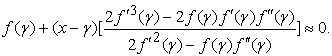From the above equation, we get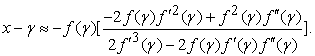(12)

Thus we can solve equation (12) by assumingas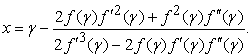(13)

This leads to the following new algorithm:

Algorithm 1.2: For given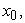compute the approximate solution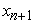by the one-step iteration scheme: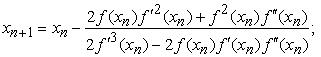(14)

for.

It will be shown that the proposed method (14) has third order convergence, and this will be done by applying the following Maple13 program:Also, based on relation (4), another new one-step iteration method can be constructed with third-order convergence. For this reason, we rewrite equation (2) as follows: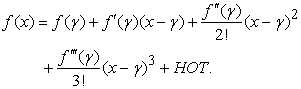(15)

Equation (15) can be approximate as(16)

Substituting (4) into the brackets of equation (16), we find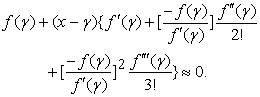That is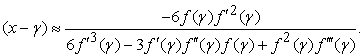(17)

Using the above equation, we can suggest the following new one-step iteration method as follows:

Algorithm 1.3: For a givencompute the approximate solutionby the one-step iteration scheme: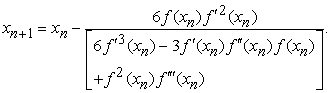It will be shown that the proposed method (18) has third order convergence, and this will be done by applying the following Maple13 program: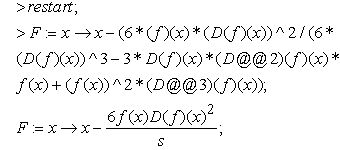where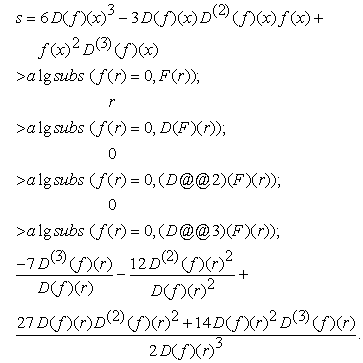3. Numerical Examples

We present some examples to illustrate the efficiency of the new proposed methods in this paper. We compare the Newton’s method (NM), the method of Saeed and Aziz  (SA), the methods of Saeed and Khthr  (SK),the method of Saeed and Khthr  (SKh) and the method proposed in this paper by the algorithms 1.1-1.3. We use the following stopping criteria for computer program:Displayed in Table 1 is the number of iterations (IT).

Table 1. Comparison of various iterative methodsDownload asPowerPoint Slide

Veiw figure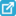View current table in a new window

4. Conclusion

We present a new method with three-order convergence for solving nonlinear equations. Analysis of efficiency and the number of iterations shows that the new algorithm is more efficient and it performs better than classical Newton’s and similar or better that the methods proposed by [8, 9, 10]. Also, we see Algorithm 1.3 diverge for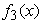becauseis a symmetric function on the interval which containReferences

  Burden, R. L. and Faires, J. D., Numerical Analysis, 9th edition, Brooks/Cole Publishing Company, 2011.In article  Chun, C., Some fourth-order iterative methods for solving non-linear equations, Appl. Math. Lett., 195, (2008), 454-456.In article  Kincaid, D. and Cheney, W., Numerical Analysis-Mathematics of Scientific Computing, Brooks/Cole Publishing Company, 1991.In article PubMed  Kou, J.; Li, Y. and Wang, X., A family of fifth-order iterations composed of Newton and third-order methods, Appl. Math. Lett., 186, (2007), 1258-1262.In article View Article  Ostrowski, A. M., Solution of Equation in Euclidean and Banach Space, 3rd edition, Academic Press, New York, 1973.In article  Porta, F. A. and Pták, V., Nondiscrete induction and iterative processes, Research Notes in Mathematics, Vol. 103, Pitman, Boston, 1994.In article  Saeed, R. K. and Ahmed, S. O., A New Fourth-order Iterative Method for Solving Nonlinear Equations, Zanco, Journal of Pure and Applied Sciences, 21(5), (2010), 1-5.In article  Saeed, R. K. and Aziz, K. M., Iterative methods for solving nonlinear equations by using quadratic spline function, Math. Sci. Lett., 2(1), (2013), 37-43.In article View Article  Saeed and Khthr, F. W., Three new iterative methods for solving nonlinear equations, Australian Journal of Basic and Applied Sciences, 4(6), (2010), 1022-1030.In article  Saeed, R. K. and Khthr, F. W., New Third-order Iterative Method for Solving Nonlinear Equations. Journal of Applied Sciences Research, 7(6), (2011), 916-921.In article  Zhou, X., Modified Chebychev-Halley methods free from second derivative, Appl. Math. Comput., 203, (2008), 824-827.In article View Article